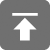# 工学1号馆

home

## Project Euler 2–Even Fibonacci numbers

Wu Yudong    January 15, 2017     欧拉计划   449

Each new term in the Fibonacci sequence is generated by adding the previous two terms. By starting with 1 and 2, the first 10 terms will be:

1, 2, 3, 5, 8, 13, 21, 34, 55, 89, …

By considering the terms in the Fibonacci sequence whose values do not exceed four million, find the sum of the even-valued terms.

1, 2, 3, 5, 8, 13, 21, 34, 55, 89, …

#include <stdio.h>
#include <string.h>
#include <ctype.h>
#include <math.h>

#define N 4000000

int a;

void solve()
{
int a, b, c, n, count = 2;
a = 1, c = 0, b = 2;
n = 3;
while (c <= N) {
c = a + b;
if (n % 2 != 0) {
a = c;
} else {
b = c;
}
n++;
if (c % 2 == 0) {
count += c;
}
}
printf("%d", count);
}

int main()
{
solve();
return 0;
}